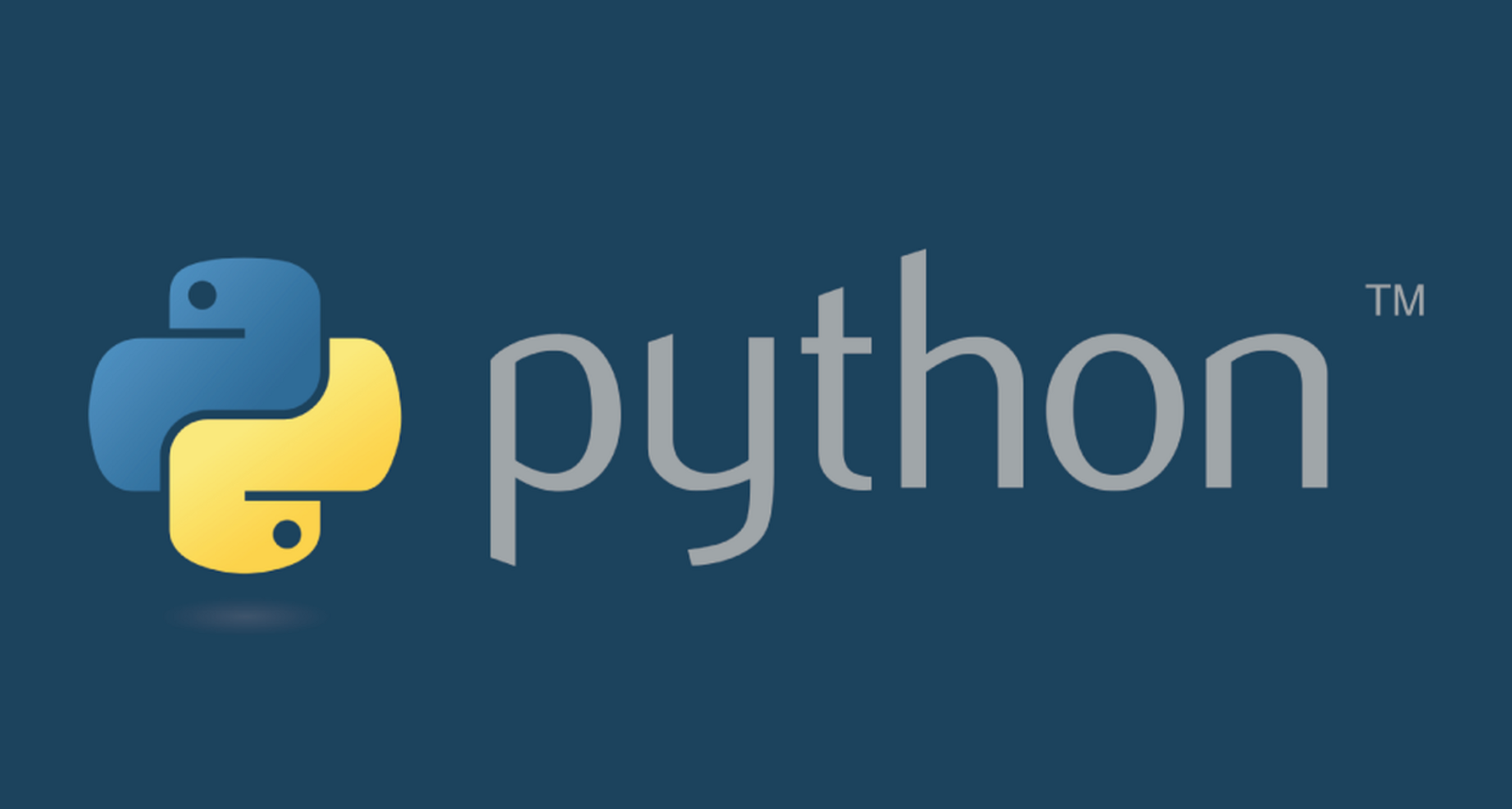Mathematics and statistics

Mathematical analysis involves a large amount of mathematical knowledge, and the mathematical knowledge involved in the data processing and analysis process can be quite complex. Therefore, it is very important to have a solid mathematical foundation. At least it is necessary to understand what is being done. It is also necessary to be familiar with the commonly used statistical concepts, because all the analysis and interpretation of the data are based on these concepts. If computer science provides data analysis tools, then statistics provide the basic concepts.

Statistics provides a lot of tools and methods for analysts, and it takes years of honing to master them all. The most commonly used statistical techniques in the field of data analysis are: 1. Bayesian method; 2. Regression; 3. Clustering; when these methods are used, it will be found that mathematical and statistical knowledge are closely combined, and both Very high demand.

Machine learning and artificial intelligence

One of the most advanced tools in the field of data analysis is the machine learning method. In fact, although data visualization and techniques such as clustering and regression are very helpful for analysts to find valuable information, in the data analysis process, analysts often need to query various patterns in the data set. These steps are very professional. Strong.

The discipline of machine learning is how to combine a series of steps and algorithms, analyze data, identify patterns in the data, find different clusters, find trends, and extract useful information from the data for data analysis. And automate the entire process.

Machine learning is becoming a fundamental tool for data analysis, so understanding its importance to data analysis is not a problem.

Data source field

Knowledge in the field of data sources is also a very important piece. In fact, although the analyst has been trained in statistics, he must also go deep into the application field and record the raw data to better understand the process of data generation. In addition, the data is not just a dry string or number, but an expression of the actual observed parameter, or more specifically its metric. Therefore, an in-depth understanding of the data source area can enhance the ability to interpret data. Of course, even for analysts who are willing to learn, it takes a lot of work to learn the knowledge of a particular field. Therefore, it is best to ask relevant experts in a timely manner.

Understand the nature of the data

The object of data analysis is naturally data. Data is a primary concern at all stages of data analysis. The raw materials to be analyzed and processed are composed of data. After processing and analyzing the data, you may end up with useful information. This information can increase the understanding of the research object, that is, the system that produces the raw data.

Data to information transformation

Data is a record of everything in the world. Anything that can be measured or classified can be represented by data. Once the data has been collected, it can be studied and analyzed to understand the nature of the thing. People often use them to make predictions, or even if they don’t make predictions, they can at least make the speculation more relevant.

When information is transformed into a set of rules that help to better understand a particular mechanism, it is said that information has been transformed into knowledge, and we can use this knowledge to predict the evolution of events.

The data can be divided into two different types: 1. The categorical type has a classification and sequencing; 2. The numerical type has discrete and continuous data; the categorical data refers to values ​​or observations that can be divided into different groups or categories. There are two types of data: class and order. There is no intrinsic order for the categories of the fixed type variables. There is no intrinsic order for the categories of the ordered variables, and the ordered variables have a pre-specified order.

Numerical data refers to values ​​or observations obtained by measurement. There are two different types of numerical data: discrete and continuous. The number of discrete values ​​is countable, and each value is distinguished from other values. Conversely, continuous values ​​result from measurements or observations whose results fall within a certain range.

Data analysis process

The data analysis process can be described in the following steps: transforming and processing raw data, presenting the data visually, and modeling for prediction. Therefore, data analysis is nothing more than the following steps, each of which plays a role in the next few steps. Therefore, data analysis can be roughly summarized as a process chain consisting of the following stages:

Problem definition, data extraction, data cleansing, data transformation, data exploration, predictive models, model evaluation/testing, results visualization and interpretation, solution deployment.